## CTSD PRECISION ADCS – PART 2CTSD architecture explained for signal chain designers

From Abhilasha Kawle

Related Vendorsless

This article will explain continuous-time sigma-delta (CTSD) ADC technology in a less traditional approach, enabling signal chain designers to envision a new class of easy to use precision ADC technology as a simple system that interconnects a few well-known components.This article will outline a step-by-step approach to building a CTSD modulator loop starting with the widely known closed-loop inverting amplifier configuration and combining it with an ADC and a DAC. Finally, the basic sigma-delta functionality from the circuit we build will be evaluated. (Source: ©Сake78 (3D & photo) - stock.adobe.com)

In Part 1, we highlighted the key challenges of incumbent signal chain designs that can be simplified significantly with a precision CTSD ADC, as it maintains continuous-time signal integrity while achieving the highest precision. Now, the question is what’s behind the CTSD architecture that enables it to achieve these advantages?

The traditional approach of explaining the concept of CTSD technology is by first understanding the basics of a discrete-time sigma-delta (DTSD) modulator loop and then substituting the discrete-time loop elements with equivalent continuous-time elements. While this method gives an in-depth understanding of sigma-delta functionality, we aim to provide a more intuitive understanding behind the inherent advantages of precision CTSD ADCs. To begin, we will outline a step-by-step approach to building a CTSD modulator loop starting with the widely known closed-loop inverting amplifier configuration and combining it with an ADC and a DAC. Finally, we will evaluate the basic sigma-delta functionality from the circuit we build.

### Step 1: Revisiting the Closed-Loop Inverting Amplifier Configuration

One of the key advantages of the CTSD ADC is that it offers an easy to drive continuous resistive input rather than a traditional switched capacitor sampler upfront. One of the circuits that has a similar input impedance concept is the inverting amplifier, which we will use as a starting block toward building a CTSD modulator loop.

A closed-loop op amp configuration has always been the go-to option for replicating an analog input with high fidelity, and Figure 1 shows one of the most popular op amp configurations, which is called an inverting amplifier configuration1. One of the measures of the fidelity is the output to input gain, also known as, in sigma-delta nomenclature, the signal transfer function (STF). Determining the parameters that affect the STF requires analyzing the circuit.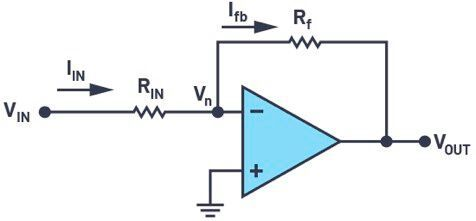Figure 1. A closed-loop op amp in inverting amplifier configuration. (Source: Analog Devices)

To refresh our mathematical skills, let’s revisit the derivation of famous VOUT/VIN. In the first step, the open-loop gain of the op amp A is assumed to be infinite. This assumption directly leads to making negative input of op amp, Vn at potential ground. The application of Kirchhoff’s laws at this node gives

Mapping this to VOUT and VIN, we get the gain or STF as in formula 2.

Next let’s go beyond the impractical assumption of infinite gain and rederive the STF with the finite gain of A for the op amp. The STF now looks like formula 3.

From here, textbooks generally describe the sensitivity toward each of the parameters RIN, Rf, and A. For our case, lets proceed toward building the CTSD loop.

### Step 2: Introducing Discretization into the Amplifier

The requirement for our ADC signal chain is a digitized version of VIN. In our next step, we introduce the digitization in this circuit. Rather than use the traditional way of putting a sampling ADC directly at the input signal, we will try a different approach and put a representative ADC that follows the amplifier output to get the digitized data. But the output of the ADC cannot be used as feedback directly, as it is required to be an analog voltage. So then, we need to follow up the ADC with a voltage digital-to-analog converter (DAC) as shown in Figure 2.

Because of the ADC and DAC, VOUT is still a representation of VIN but with quantization error due to the digitization added. So, nothing has changed in the signal flow from VIN to VOUT. One point of note here, to keep the functionality of loop symmetric about 0 V and ease our mathematical derivation, the references of ADC and DAC are chosen to be as in formula 4.

### Step 3: Introducing the Analog Accumulator–The Integrator

Is the closed-loop configuration in Figure 2 stable? Both the ADC and DAC are discretization elements working on a sampling clock, MCLK. It has been an unachievable dream of converter specialists to design a delay free ADC or DAC. Since these loop elements are clocked, the input is generally sampled on one edge and processed on the other clock edge. So, the output of the ADC and DAC combination VOUT, which is the feedback in Figure 2, is available only after 1 clock cycle delay.

Does this delay in feedback have any implication on stability? Let’s trace how VIN transfers along. For simplification let’s assume VIN = 1, RIN = 1, Rf = 1, and the gain of op amp A is 100. At the first clock cycle, the input voltage is 1 and the DAC output feedback, VOUT or VOUTDAC, is 0 and is not available until the next clock edge. As we trace the error between the input and feedback to the output of the amplifier and ADC, we can see the output keeps growing exponentially and this is technically termed as the runaway problem.

### Subscribe to the newsletter now

#### Don't Miss out on Our Best Content

Unfold for details of your consent

This happened because the ADC input works on instantaneous error gained up by the amplifier; that is, the ADC decides even before the feedback is available, which was not required. If the ADC works on an accumulated, averaged error data so that the error due to 1 clock delay of feedback is averaged out, then the output of system would be bounded.

The integrator is one such analog equivalent of an averaging accumulator. The gain of the loop is still high but only at low frequencies or, in other words, in the frequency bandwidth of interest. This ensures that the ADC is not presented with any instantaneous errors that can lead to a runaway situation. So, the loop is now amplifier modified as an integrator followed by the ADC and DAC, as shown in Figure 3a.

### Step 4: Simplifying the Feedback Resistor

Our element of interest is DOUTADC, so let’s rearrange the loop elements to highlight DOUTADC as the output of the system, as shown in Figure 3b. Next, let’s visit the simplification of the DAC and Rf path. And for that let’s dig into the DAC’s details. The purpose of the DAC is to convert a digital code, DIN, to an equivalent analog current or voltage in proportion to the reference. To further extend the advantages of continuity to reference, what we have considered here is a general DAC architecture based on a resistor ladder that has no switching load on reference. Let’s review a thermometric resistor DAC,2 which converts DIN to the DAC current, with relation to Equation 5.

Where VREF = VREFP – VREFM, the total reference voltage across the DAC.

• DIN = Digital input in the thermometric code
Rf = Feedback resistor; split as each unit element
N = Number of bits

To get the voltage output, an I to V conversion follows by using an op amp in a transimpedance configuration,3 as shown in Figure 4.

So, there is formula 6.

Going back to our discretized loop of Figure 3b, this VOUTDAC is again converted back to current, Ifb, through the feedback resistor of the inverting amplifier, implying the signal flow is IDAC → VOUTDAC → Ifb. Mathematically, see formula 7.

From the above signal flow and formula, we see that converting VOUTDAC to Ifb is a redundant step that can be bypassed. Removing the redundant elements and, for simplicity, representing (VREFP – VREFM) as VREF, let’s redraw our loop, as shown in Figure 5.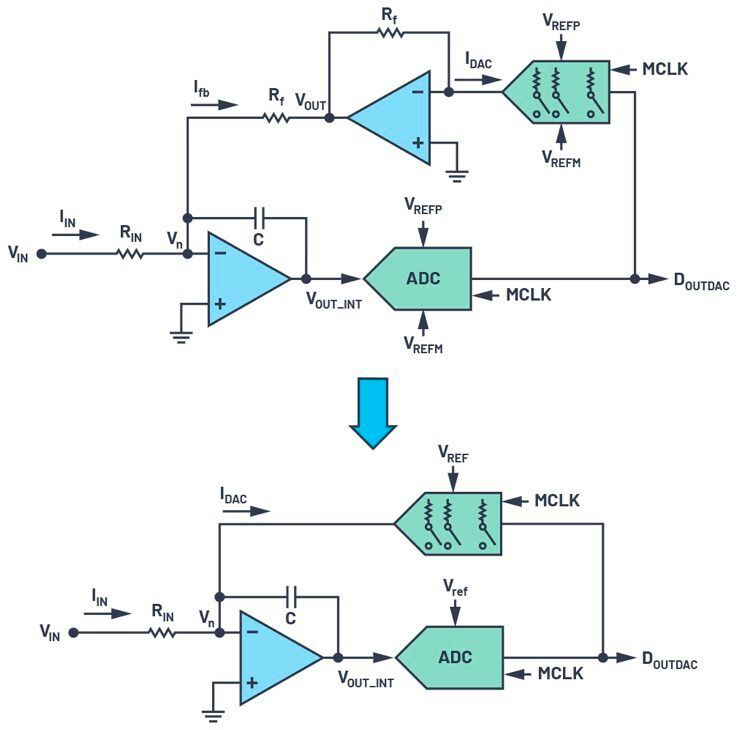Figure 5. Removing redundant I to V and the feedback resistor. (Source: Analog Devices)

And voila! We have built a first-order sigma-delta loop! And all by stitching together well-known elements—an inverting amplifier, an ADC, and a DAC.

### Step 5: Understanding Oversampling

We have up to now grasped the construction of a CTSD loop, but we have yet to appreciate the particularities offered by this fanciful loop. The first step toward that is understanding oversampling. ADC data is useful only if there are enough sampled and digitized data points to extract or interpret the analog signal information. The Nyquist theorem advises that, for faithful reconstruction of an input signal, the sampling frequency of the ADC should be at least twice the frequency of the signal. If we keep adding more data points over this minimum requirement, the error in interpretation would be further reduced. Following this line of thought, in sigma-delta the sampling frequency is selected to be much higher than the suggested Nyquist frequency and this is known as oversampling. Oversampling4 helps reduce the quantization noise in the frequency band of interest by spreading the total noise over much higher frequency, as shown in Figure 6.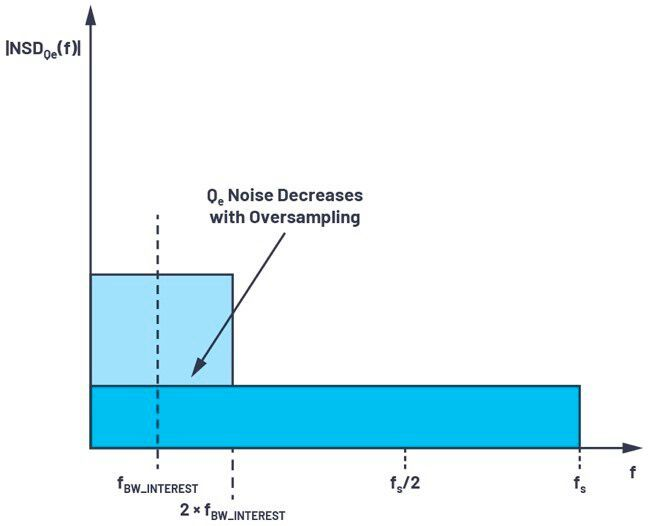Figure 6. A noise spectral density comparison between Nyquist sampling and oversampling. (Source: Analog Devices)

### Step 6: Understanding Noise Shaping

Signal chain designers shouldn’t feel lost when sigma-delta experts use terms like noise transfer function (NTF) or noise shaping,4 and our next step will help them get an intuitive understanding of these terms as they are unique to sigma-delta converter nomenclature. Let’s revisit our simple inverting amplifier configuration and introduce the error Qee at the output of the amplifier, as shown in Figure 7.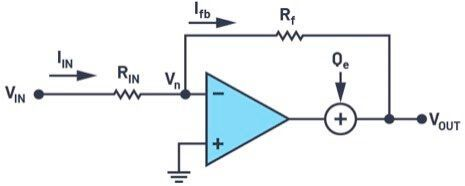Figure 7. The introduction of an error in an inverting amplifier configuration. (Source: Analog Devices)

The contribution of this error at the output is quantified as seen in formula 8.

The mathematical formula translates that the error Qe is attenuated by the open-loop gain of the amplifier, which is just reiterating the advantage of a closed loop.

This understanding of the closed-loop advantage can be extended to quantization error Qe of the ADC in CTSD loop, which is the error introduced due to digitization of the continuous signal at the output of the integrator, as shown in Figure 8.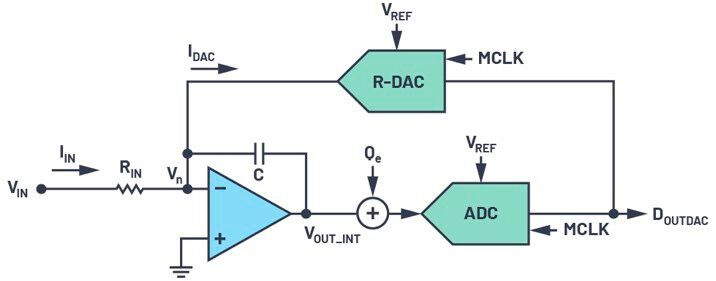Figure 8. The introduction of quantization error Qe in a sigma-delta loop. (Source: Analog Devices)

We can now intuitively conclude that this Qe would be attenuated by the integrator. The integrator TF is |HINTEG (f)|= 1/|s × RC| = 1/2πfRC and its corresponding frequency domain representation is shown in Figure 9. Its profile is equivalent to a low-pass filter profile with high gain at low frequencies, and the gain reduces linearly as frequency increases. Correspondingly, the attenuation for Qe would then look like a high-pass filter.

The mathematical representation of this attenuation factor is the noise transfer function. For an interim, let’s ignore the sampler in the ADC and the switches in the DAC. The NTF, VOUTADC ⁄ Qe, can be evaluated by following the same exercise as we did for the inverting amplifier configuration, which in the frequency domain looks like a high-pass filter profile, as shown in Figure 10.

In the frequency band of interest, the quantization noise is completely attenuated and pushed to “not to our concern” high frequencies. This is what is called noise shaping.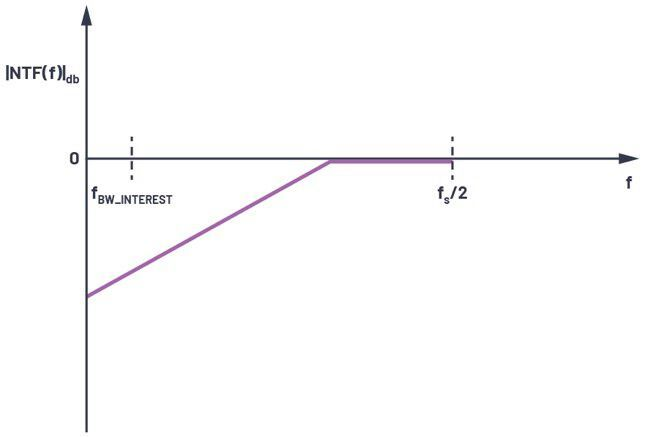Figure 10. Noise transfer function without the sampler—has a high-pass filter profile. (Source: Analog Devices)

With the sampler in loop, the quantization noise shaping analogy remains the same. The difference being the NTF frequency response would have replicated images at every multiple of fs, as shown in Figure 10, thus creating notches at every integer multiple of the sampling frequency.

The uniqueness of sigma-delta architecture lies in the fact that putting an integrator and a DAC loop around a crude ADC—for example, a 4-bit ADC—and applying the concept of oversampling and noise shaping reduces the quantization noise significantly in the frequency bandwidth of interest and masks this crude ADC to a 16- to 24-bit precision ADC.

These basics of the first-order CTSD ADC can now be extended to any order of modulator loop. The sampling frequency, the crude ADC specifications, and the order of loop are top-level design decisions driven by the performance requirements of the ADC.

### Step 7: Completing the CTSD Modulator with a Digital Filter

Generally, in an ADC signal chain, the digitized data is postprocessed by an external digital controller for any signal information extraction. In sigma-delta architecture, as we know now, the signal is oversampled. If this oversampled digital data is directly given to the external controller, then there is a lot of redundant data that needs to be processed. This causes excess power and real estate cost overheads in the digital controller design. So, before data is presented to the digital controller, the data samples are dropped in an efficient way without affecting the performance. This process is called decimation and is done by digital decimation filters. Figure 11 shows a typical CTSD modulator with on-chip digital decimation filters.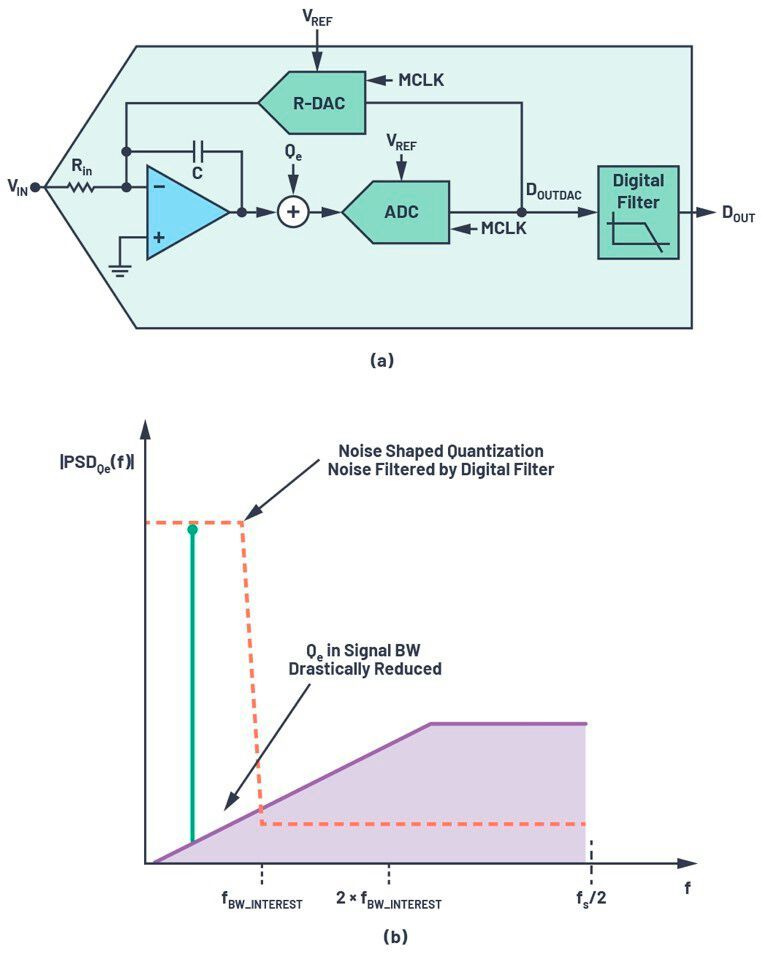Figure 12. (a) A block diagram of a CTSD ADC modulator loop from an analog input to a digital output. (b) A frequency spectrum representation of an input signal at the output of a modulator and the output of a digital filter. (Source: Analog Devices)

Figure 12b shows frequency response for an in-band analog input signal. At the output of the modulator we observe the noise shaping of the quantization noise, drastically reducing it in frequency band of interest. The digital filter helps attenuating the shaped noise beyond this frequency bandwidth of interest so that at the final digital output, DOUT, is at the Nyquist sampling rate.

### Step 8: Understanding the Clock Sensitivity of CTSD ADCs

So far, we have understood how CTSD ADCs keep the continuous integrity of the input signal, which significantly simplifies signal chain design. There are also a few limitations with this architecture, mainly dealing with the sampling clock, MCLK. The CTSD modulator loop works on the concept of integrating the error current between IIN and IDAC. Any error in this integrated value would cause the ADC in loop to sample the error and would reflect this in the output. For our first-order integrator loop, the integrated value over the sampling time period of Ts for constant IIN and IDAC is given by formula 10.

For an input of 0, the parameters that would affect this integration error are

• MCLK frequency: As indicated by Equation 10, if the MCLK frequency scales, then the RC coefficient that controls the slope of integration also needs to be retuned to get back the same integrated value. This implies that a CTSD modulator is tuned for a fixed MCLK clock frequency and cannot support varying MCLK.
MCLK jitter: The DAC code and, hence, IDAC change every clock time period Ts. If the IDAC time period randomly changes, then the average integrated value keeps changing, as shown in Figure 13. So, any error in the sampling clock time period in the form of jitter would affect the performance of the modulator loop.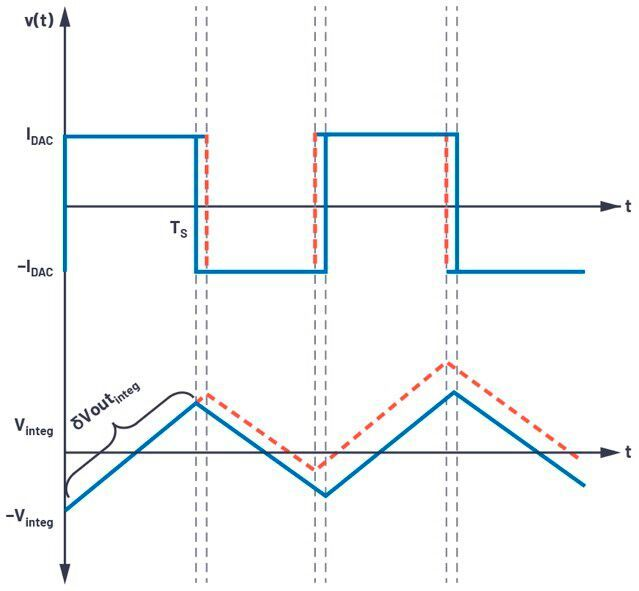Figure 13. The clock sensitivity of a CTSD modulator. (Source: Analog Devices)

### Step 9: Voila! All Set to Explain the CTSD Concept to Your Buddies!

Part 1 highlighted certain signal chain advantages of a CTSD ADC, while Part 2 focused on the insights of the modulator loop built from Step 1 to Step 6 using the concept of a closed-loop op amp configuration. Figure 11a also helped us visualize these advantages.

The input impedance of a CTSD ADC is equivalent to the input impedance of the inverting amplifier, which is resistive and easy to drive. Using innovative techniques, the reference used by the modulator loop’s DAC has also been made resistive. The sampler of the ADC is after the integrator and not directly at the input, which enables inherent alias rejection for interferers outside the frequency band of interest. We will deep dive into each of these advantages and their corresponding impact in a signal chain in the next few articles of this series. In the next article, we’ll begin with the most unique advantage: inherent alias rejection. Keep an eye out for Part 3 to catch up on inherent alias rejection and to learn more about its quantification using a new set of measurements and performance parameters introduced for the first time with the AD4134, which is based on the CTSD architecture.

### References

1 Hank Zumbahlen. “Mini Tutorial MT-213: Inverting Amplifier.” Analog Devices, Inc., February 2013.
2 Walt Kester. “MT-014 Tutorial: Basic DAC Architectures I: String DACs and Thermometer (Fully Decoded) DACs.” Analog Devices, Inc., 2009.
3 Luis Orozco. “Programmable-Gain Transimpedance Amplifiers Maximize Dynamic Range in Spectroscopy Systems.” Analog Dialogue, Vol. 47, No. 2, May 2013.
4 Walt Kester. “MT-022 Tutorial: ADC Architectures III: Sigma-Delta ADC Basics.” Analog Devices, Inc., 2009.
5 Pawel Czapor. “Sigma-Delta ADC Clocking—More Than Jitter.” Analog Dialogue, Vol. 53, No. 3, April 2019.
Pavan, Shanthi, Richard Schreier, and Gabor C. Temes. Understanding Delta-Sigma Data Converters, 2nd edition. Wiley, January 2017.

### Acknowledgements

The author would like to thank Praveen Varma and Roberto Maurino for their helpful insights in putting together this simplified way of explaining the CTSD ADC technology.

* Abhilasha Kawle is Analog Design Manager at Analog Devices.

(ID:47957625)